June 01, 2020

## Introduction

Each application needs to store information temporarily or permanently, for example, the data of a person, the measurements were taken on a machine, the detail of sales made in a warehouse, etc., for this the programming languages ​​have provided data containers that allow the information to be stored and processed according to some criteria. These containers are called data structures and can be classified into `primitive` and `complex` data structures: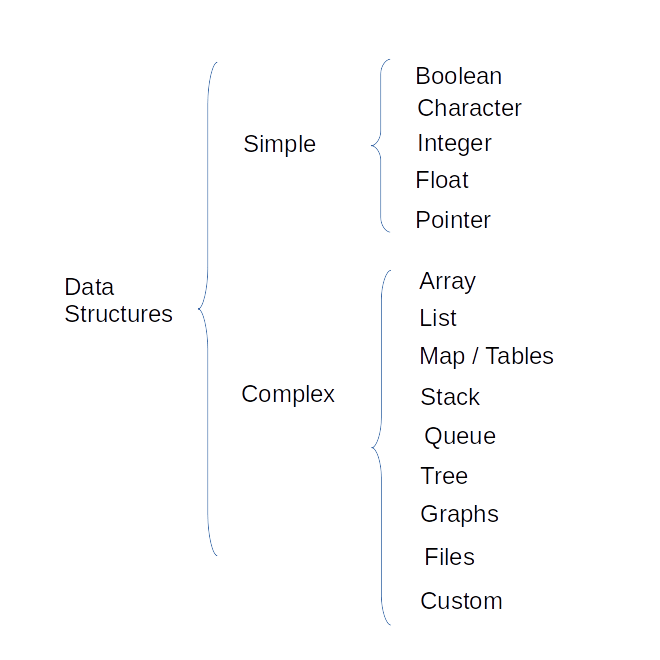Image 1: Data Structures

In this document, we are going to focus on complex structures.

## Arrays

An array is a container of predefined size n, whose elements are in contiguous memory spaces and its indexes are between ranges `0` and `n-1`, its uses are mainly:

• Temporary storage
• As IO routine buffers
• Sending data as arguments in method parameters
• Data return in methods
• Randomic access

For example an array of `4 integer elements` whose values ​​are: `9`, `5`, `-2`, `7` can be represented as follows: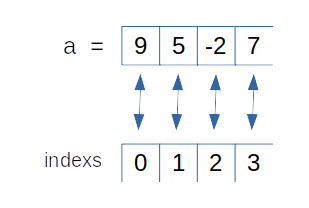Image 2: Array

Each element is accessed through its `index` which starts at `0`, for example the last value (`7`) can be accessed by index 3 like this: `a => 7`

The definition of an array is very similar between programming languages, for example:

Golang:

``````a := int{9,5,-2,7}
``````

Java:

``````int[] a = {9,5,-2,7};
``````

Arrays can have several dimensions, but more than three dimensions can make it difficult to read a program and can generate errors. A two-dimensional arrangement can be defined as follows:

Golang:

``````b := int { {5,3,7},{9,7,8},{0,-3,-3} }
``````

Java:

``````int [][] b = { {5,3,7},{9,7,8},{0,-3,-3} };
``````

Each language provides several alternatives to declare arrays, however, it is always necessary to define the maximum size `n` of it.

Golang

``````// The array is defined by its size n
var c [n]int

// It is possible to define the values ​​assigned to each index
c := [n]int{index i:value, index j:value}
``````

Java

``````// The array is defined by its size n
int[] c = new int[n];

// The array is defined by the assigned elements
int[] c = {value 1, value n};

// The array is defined and initialized by its values
int[] c = new int[]{value 1, value n};
``````

### Basic operations

In an arrangement we can find the following operations:

• `get`.- get the value of an element in an index
• `size`.- get the size of an array

### Most obvious problems

The main drawbacks with using arrays are:

• The size of an array is defined in its creation and cannot be modified.
• In Golang by default passing an array to a function as a parameter passes the copy of the array, which can be slow if the array is large.

To solve these problems, programming languages ​​have provided certain structures that allow them to be dynamically modified, such as: `Slices`, `Maps` in the case of Golang or `ArrayList` and `Vector` in the case of Java, among others.

### Implementations

Golang / Slices

Its main characteristics are:

• It is a structure that allows to increase or decrease its size dynamically.
• They are implemented using arrays internally.
• Passed by reference to functions which means that there is no need to make a copy of the entire array since only the address of the same is sent.
• It is not synchronized.

A Slice can be declared in the following way:

``````// The slice is defined by the assigned elements
c := []int{value 1, value n}

// The compiler identifies the length of the array based on its
// elements
c := [...]int{value 1, value n}

// The make function allows the creation of an empty
// slice with the length defined by n
c := make([]int , n)
``````

Its main properties are: `capacity` and `length`, the length of a slice is the same as the length of an array and can be found using the `len()` function, the capacity can be obtained using the `cap()` considering that Go automatically doubles the current length every time it runs out of space to store new items, this can be inconvenient if it is a large slice as it may take up more memory than expected.

Java / ArrayList

In the case of Java, it is possible to generate dynamic arrays using the `ArrayList` class, which is an implementation of the `List` interface. Its main characteristics are:

• It is a structure that allows to increase or decrease its size dynamically.
• Internally it uses an Array to store its elements which allows returning its elements by their index.
• Allow duplicate and null values.
• Maintains the insertion order of its elements.
• It is not synchronized.

An ArrayList can be declared in the following way:

``````// Defining a dynamic list of Strings
List<String> c = new ArrayList<String>();
``````

The length of an `ArrayLis` can be obtained using the `size()` method while its capacity automatically increases starting with a value of `10`.

In the case of lists, the most obvious difference is that its elements are not found in contiguous spaces of memory, however each element (known as a node) knows the location of its next value, one of the main advantages of Lists over Arrays is that lists allow you to dynamically change their size, allowing you to increase or decrease their size according to your needs.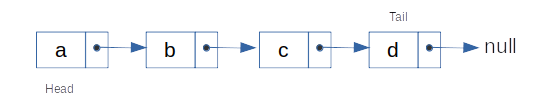Image 3: Lists

Each node uses at least two memory spaces, one to store the current data and the other to store a pointer to the next element, thus creating a sequence of elements that generate the list. The first node in the list is known as the `head` while the last node is called the `tail`.

The use of linked lists has great advantages: they are easy to understand and implement, they are generic enough to be used with more complex structures and the search process in them is fast, however, once a node is reached, it is not possible to directly reach the previous node, in order to solve this, it is possible to use an implementation called Doubly Linked Lists which allow solving this problem.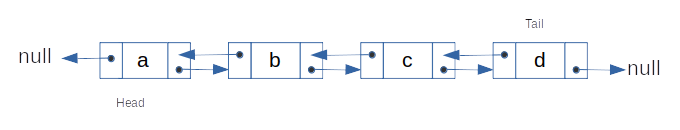Image 4: Doubly Linked Lists

### Implementations

Golang

The implementation of a linked list requires the creation of a structure that represents a node, this structure must contain a value and a pointer to the following element:

``````type Node struct {
Value int
Next *Node
}
``````

From this structure, its possible define operations such as:

• `addNode`
• `searchNode`
• `deleteNode`
• `traverse`
• `size`

For example addNode can be defined as follows:

``````func addNode(t *Node, v int) int {
if root == nil {
t = &Node{v , nil}
root = t
return 0
}

if v==t.Value {
return -1
}

if t.Next==nil {
t.Next = &Node{v,nil}
return -2
}

}
``````

Golang provides an implementation for Double Linked Lists structures, this implementation can be found in the “list” package, an implementation example is:

``````package main

import (
"container/list"
"fmt"
)

func main() {
// Create a new list and put some numbers in it.
l := list.New()
e4 := l.PushBack(4)
e1 := l.PushFront(1)
l.InsertBefore(3, e4)
l.InsertAfter(2, e1)

// Iterate through list and print its contents.
for e := l.Front(); e != nil; e = e.Next() {
fmt.Println(e.Value)
}
}
``````

Java

The implementation of lists in Java is similar to the one carried out in Golang, in case you need to carry out your own implementation we can generate something similar to the following:

``````class Node {
int value;
Node next;
}
``````

In the case of the method addNode the implementation can be similar to the following:

``````public int void addNode(Node t, int v) {
if root == null {
t = new Node( v , null );
root = t;
return 0;
}

if v==t.value {
return -1
}

if t.next==null {
t.next = new Node( v , null );
return -2
}

}
``````

In the case of Java the list implementations are: AbstractList, AbstractSequentialList, ArrayList, AttributeList, CopyOnWriteArrayList, LinkedList, RoleList, RoleUnresolvedList, Stack, Vector, with LinkedList being a doubly linked List implementation.

An example of using the LinkedList class is:

``````import java.util.*;

{
public static void main(String args[])
{

System.out.println("Users : " + users);
}
}

// Output: Users : [Alejandro, Edison, Mercedes, Patricio, Ximena]

``````

## Map / HashTables

Maps and HashTables are equivalent `key-value` structures, their main characteristic being the use of an identifier (key) which will have a value associated with the condition that this key must be unique and differentiated from other keys.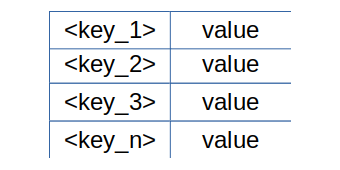Image 5: Map

The performance of this structure depends on the following factors:

• Hash Function
• Size of HashTable
• Collision handling method

### Implementations

Golang / Map

Go does not exclude any type of data to be used as a key, the only condition being that key values ​​can be differentiated from each other. Your definition must follow this format:

``````<variable_name> := make(map[key]value_type)

// Example:
students := make(map[string]string)
students["1"] = "Carla Flores"
students["2"] = "Marco Salazar"
students["3"] = "José Navas"
``````

This structure is faster and more versatile than arrangements and slices, being very convenient to use where a key/value structure is warranted.

Java / Map

In Java, Map is an interfaz of key-value type which in turn is implemented by several classes such as: HashMap, HashTable, LinkedHashMap, TreeMap among others. In the particular case of HashMap the use of null values ​​is allowed as well as null keys, this class is equivalent to Hashtable except that it is not synchronized. It is important to indicate that the order of its elements is not guaranteed to remain constant over time, it should be noted that the objects used as keys in a Hashtable must implement the hashCode and equals method.

``````// A map is defined whose keys will be of type String and its values
//​​ of type Strings.
Map<String,String> map = new HashMap<String,String>();

// The definition of a hashTable is similar to that of a hashMap with
// the difference that a hashTable is synchronized which means that a
// thread-safe implementation is not necessary.
Map<String,String> table = new Hashtable<String,String>();
``````

## Stacks

The main characteristic of a stack is that its elements use for its processing the technique known as LIFO (Last In First Out), which means that the element that is stored at the end will be the first element to be obtained by the stack.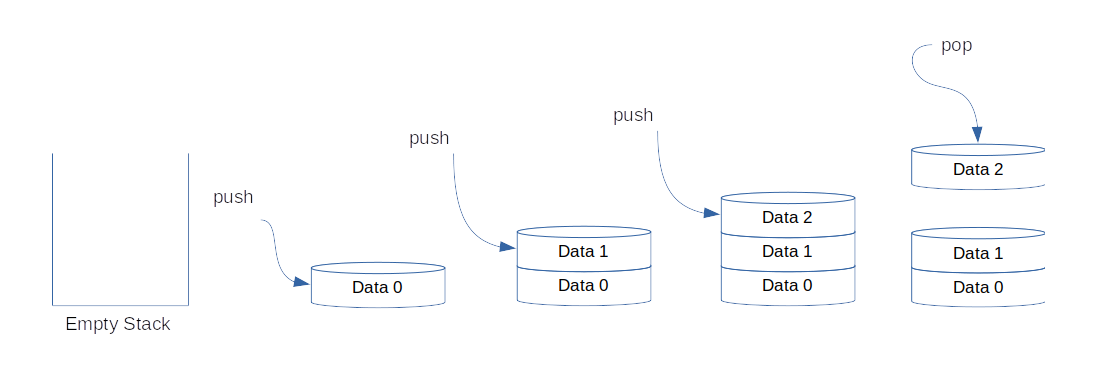Image 6: Stack

### Basic Operations

The basic operations carried out on batteries are:

• `Push`: insert an element in the stack header
• `Pop`: get the first item by removing it from the stack (LIFO)
• `isEmpty`: returns true if the stack is empty
• `Top`: return the first item in the stack without removing it

### Implementations

Golang

The implementation of a Stack in Golang is similar to the implementation of a queue with the difference that the Stacks use the LIFO (Last In First Out) storage method.

A possible implementation of Stack methods can be:

``````func Push(v int) bool {
if stack == nil {
stack = &Node(v,nil)
size = 1
return true
}

temp := &Node(v,nil)
temp.Next = stack
stack = temp
size++
return true
}

func Pop(t *Node) (int, bool) {
if size==0 {
return 0,false
}

if size==1 {
size = 0
stack = nil
return t.Value, true
}

stack = stack.Next
size--
return t.Value,true
}

func traverse(t *Node) {
if size == 0 {
fmt.Println("Empty Stack!")
return
}

for t != nil {
fmt.Printf("%d -> ", t.Value)
t = t.Next
}
fmt.Println()
}
``````

Java

The implementation of stack methods in java can be done in the following way:

``````public boolean push(int v) {
if( stack==null ){
stack = new Node(v,null);
size = 1;
return true;
}

temp = new Node(v,null);
temp.Next = stack;
stack = temp;
size++;
return true;
}

public int pop(Node t) {
if( size==0 ){
return 0;
}

if( size==1 ){
size = 0;
stack = nil;
return t.Value;
}

stack = stack.Next;
size--;
return t.Value;
}

public void traverse(Node t) {
if( size == 0 ) {
System.out.println("Empty Stack!");
return;
}

while( t != null ) {
System.out.println("%d -> " + t.Value);
t = t.Next;
}
}
``````

Java provides the Stack implementation that contains the necessary methods for the process in LIFO form.

## Queues

The queues follow the FIFO (First In First Out) mechanism to store and obtain their values, this means that the first values ​​to be inserted in a queue will be the first elements to be returned.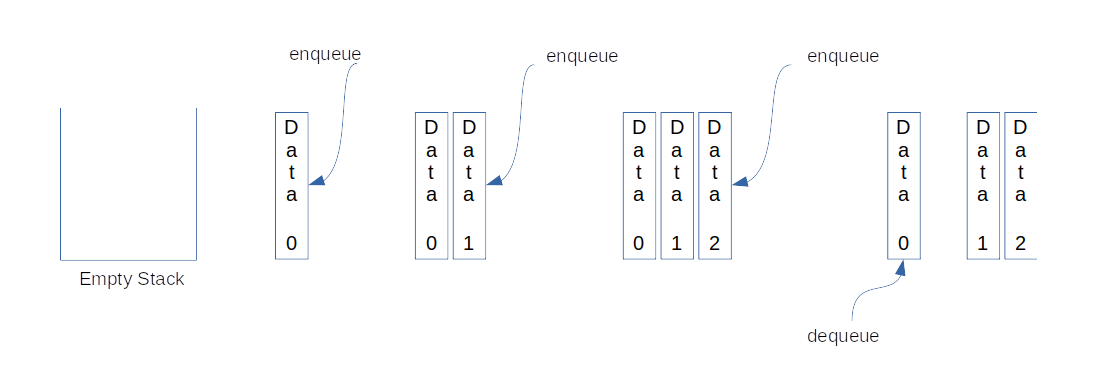Image 7: Queue

### Basic Operations

The basic operations carried out on queues are:

• `Enqueue`: insert an element at the end of the queue
• `Dequeue`: remove an item from the start of the queue (FIFO)
• `isEmpty`: returns true if the queue is empty
• `Top`: returns true if the queue is empty

### Implementations

Golang

The implementation of a queue in Golang requires the definition of a node type structure and the push and pop methods mainly.

``````type Node struct {
value int
next *Node
}

func Push(t *Node, v int) bool {
if queue==nil {
queue = &Node(v, nil)
size++
return true
}

t = &Node{v,nil}
t.Next = queue
queue = t
size++

return true
}

func Pop(t *Node) (int, bool) {
if size==0 {
return 0, false
}

if size==1 {
queue = nil
size--
return t.Value, true
}

temp := t
for (t.Next) != nil {
temp = t
t = t.Next
}

v := (temp.Next).Value
temp.Next = nil

size--
return v, true
}
``````

From the existing structures in Golang it can be highlighted that the implementation “container/list” can solve the use of queues.

Java

The implementation of the previous algorithm (queues) can be done as follows:

``````class Node {
int value;
Node next;
}

public boolean push(Node t, int v) {
if( queue==null ){
queue = new Node(v, null);
size++;
return true;
}

t = new Node(v,nil);
t.Next = queue;
queue = t;
size++;

return true;
}

public int pop(Node t) {
if( size==0 ){
return 0;
}

if( size==1 ){
queue = nil;
size--;
return t.Value;
}

temp = t;
while( t.Next ) {
temp = t;
t = t.Next;
}

int v = (temp.Next).Value;
temp.Next = null;

size--;
return v;
}
``````

Java provides queue implementations through the classes that implement the Queue interface such as:

• `AbstractQueue`
• `ArrayBlockingQueue`
• `ArrayDeque`
• `ConcurrentLinkedDeque`
• `ConcurrentLinkedQueue`
• `DelayQueue`
• `LinkedBlockingDeque`
• `LinkedBlockingQueue`
• `LinkedList`
• `LinkedTransferQueue`
• `PriorityBlockingQueue`
• `PriorityQueue`
• `SynchronousQueue`

Who provide the necessary methods to add and get nodes to a queue.

## Trees

A tree is a hierarchical structure that consists of the union of vertices (nodes), used as storage in complex algorithms in various fields such as indexes for storage in databases, Artificial Intelligence, among others.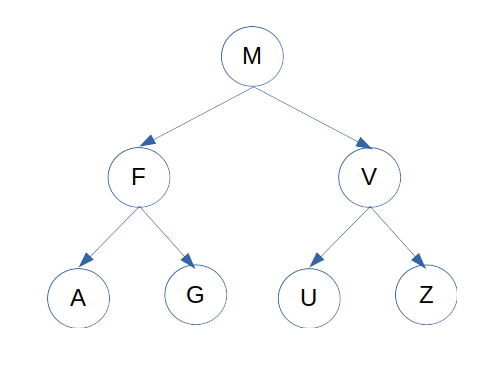Image 8: Trees

For its operation, the trees handle a certain thermology that is important to define:

• Root: root node of the entire structure. Example: `M`
• Parent: node from which new hierarchies emerge. Example: `M`, `F`, `V`
• Child: node that is in a lower hierarchy than another. Example: `F`, `V`, `A`, `G`, `U`, `Z`.
• Leaf: node from which no hierarchy follows. Example: `A`, `G`, `U`, `Z`.
• Sibling: nodes that are on the same level. Example: `F`, `V`, `A`, `G`, `U`, `Z`.

There are several types of trees which have a specific use, among the common ones we have:

• Balanced trees: is a type of tree in which its leaves are at a certain distance from its root node.
• Binary trees: is a type of tree in which each node has a maximum of two child nodes.
• Binary search trees (BST): in this type of tree are the following properties:
• The nodes in the left branch contain only nodes whose keys are less than the node from which it was split.
• The nodes of the right branch contain only nodes whose keys are greater than the node from which it was split.
• Each node in turn is of type BST.
• AVL Trees: is a self-balancing BST tree, this implies that the height of its subtrees at any node differs up to one, otherwise it is necessary to balance the tree again to achieve this property.
• Red Black Trees: it is a type of self-balanced BST tree with the difference that each node of the binary tree has an extra bit that represents the color of the node, this color is used to ensure that the tree remains balanced during the insertion and removal processes.
• Trees 2-3: in this case each node has two children (two nodes) and one data element or three children (three nodes) and two data elements.
• Trie: is a type of tree used especially in the efficient search and storage of character strings, each node stores the possible characters of an alphabet.

### Implementations

Golang

``````type Tree struct {
Left *Tree
Value int
Right *Tree
}

func traverse(t *Tree) {
if t == nil {
return
}

traverse(t.Left)
fmt.Print(t.Value, " ")
traverse(t.Right)
}

func create(n int) *Tree {
var t *Tree
rand.Seed(time.Now().Unix())
for i:=0; i < 2*n; i++ {
temp := rand.Intn(n * 2)
t = insert(t, temp)
}
return t
}

func insert(t *Tree, v int) *Tree {
if t == nil {
return &Tree{nil, v, nil}
}
if v == t.Value {
return t
}
if v < t.Value {
t.Left = insert(t.Left, v)
return t
}
t.Right = insert(t.Right, v)
return t
}
``````

Java

``````class Tree {
Tree left;
int value;
Tree right;
}

public void traverse(Tree t) {
if(t == null ){
return;
}

traverse(t.left);
System.out.println(t.Value + " ");
traverse(t.right);
}

public Tree create(int n) {
Tree t = null;
Random rand = new Random();

for(int i=0; i < 2*n; i++) {
int temp = rand.nextInt(n * 2);
t = insert(t, temp);
}
return t;
}

public Tree insert(Tree t, int v) {
if( t == nil ) {
return new Tree(null, v, null);
}
if( v == t.Value ) {
return t;
}
if( v < t.Value ) {
t.Left = insert(t.Left, v);
return t;
}
t.Right = insert(t.Right, v);
return t;
}
``````

## Graphs

A graph is a set of nodes connected in the form of a network, its nodes are called vertices and a pair (x, y) is called edge which indicates that vertex x is connected to vertex y. A border can contain weight and cost, which represents the effort required to traverse from one node to another.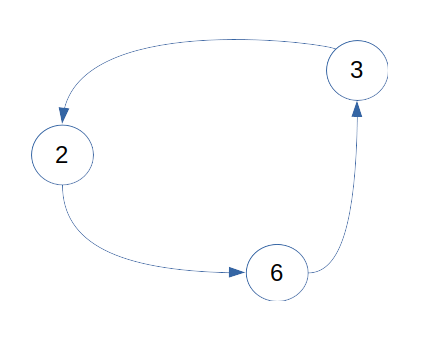Image 9: Graphs

Graphs can be of type:

• Cyclic: it is a graph where all or a number of vertices are connected forming a chain.
• Acyclic: in this case there is no closed chain.
• Directed: it is a graph with edges that have an associated direction.
• Acyclic Targets: is a directed graph that has no cycles in it.

## Files

This structure is a collection of records that can be obtained or stored in secondary storage devices, in general this type of structure is used once has been a previous process where other data structures are involved, for example, the student registration process or the payment of fees for the purchase in a commercial entity, that process generally result in storing this information on files.

Each language has implemented different ways of processing files, for example Golang has defined in the package “os” and “io” functions that allow open, read and store information, for example a file can be opened using the Open function:

``````file, err := os.Open("file.txt")
if err != nil {
log.Fatal(err)
}
defer file.Close()

fmt.Print(b)
``````

Similarly in Java you can have the following:

``````try {
File file = new File("file.txt");
FileInputStream fis = new FileInputStream(file);

System.out.println("file content: ");

int r = 0;
while ((r = fis.read()) != -1) {
System.out.print((char)r);
}

} catch (Exception e) {
e.printStackTrace();
}
``````

## Custom Structures

This type of structures are created by the developer responding to the need needs to be modeled and implemented, it can be composed of other simple and complex structures without there being a real limit in terms of the number of elements allowed in them. Each element of the structure is called a field or attribute depending on the type of language used, but finally it represents a characteristic of the modeled entity, for example, a person can be defined by its main attributes such as: height, weight, eye color, name, gender, city of birth, countries you have visited, etc.

Each language has implemented a way of handling this type of structure, so for example in the case of Golang you can use the struct type to create custom data types, for example:

``````type employer struct {
name string
id     string
}

type person struct {
heigh int
weigh int
eye_color string
name string
family map[string]persona
countries_visited []string
}
``````

A structure, in turn, can be made up of other structures, which allows the modeled entity to be represented in a real way, for example, the person attribute has been defined as a map whose key is of type string and where an alphanumeric id could be stored by example and whose value is a person type entity, this would allow to represent the members of a family. Another similar attribute in the workplace, which in this case allows you to store an entity of the employer type if it exists or nil (null value) if it does not exist.

The representation of structures in particular to the language, for example in the case of Java the use of classes is necessary to reproduce the same effect:

``````class Employer {
String name;
String id;
}

class Person {
int height;
int weight;
String eyeColor;
String name;
Map<String,Person> family;
Employer workPlace;
List<String> countriesVisited;
}
``````

The API’s and complete code examples can be found on the following resources: